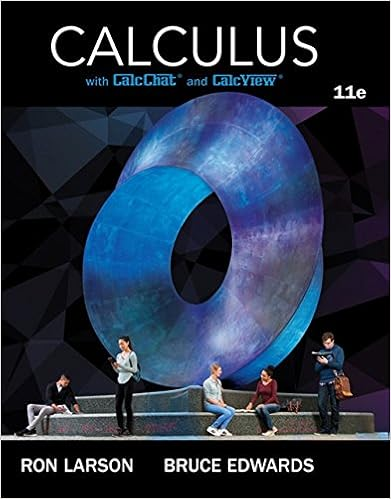# Assignment5Solutions - MAT224 Assignment 5 Solutions 1(a...

• Homework Help
• 4
• 100% (2) 2 out of 2 people found this document helpful

This preview shows page 1 - 2 out of 4 pages.

##### We have textbook solutions for you!
The document you are viewing contains questions related to this textbook.The document you are viewing contains questions related to this textbook.
Chapter 14 / Exercise 12
Calculus
Edwards/LarsonExpert Verified
MAT224 Assignment 5 Solutions1.(a) LetAbe a symmetric2×2matrix with positive eigenvalues. Show thathx, yi=xtAy,defines an inner product onR2. (For instance, ifA=2-1-11, thenh11,01i=(11)2-1-1101=0.
(b) LetA=2-1-11. Leth,ionR2be defined byhx, yi=xtAy. (By Part (a) this is an inner product onR2.) Show thatT:R2R2defined byT(x) =-2322xis symmetric with respect toh,i.Solution:(a) First, let us verifylinearity in the first variable(i.e. Condition (i) in the definition). Givenx, y, zR2andcR,hcx+y, zi= (cx+y)tAz= (cxt+yt)Az=cxtAz+ytAz=chx, zi+hy, zi,as desired. As for symmetry (i.e. Condition (ii) in the definition), we havehx, yi=xtAy(*)= (xtAy)t=ytAt(xt)t=ytAx=hy, xi.Note that(*)holds becausextAyis a1×1matrix (i.e. a number).Conditions (i) and (ii) imply linearity in the second variable as well, forhx, cy+zi(ii)=hcy+z, xi(i)=chy, xi
##### We have textbook solutions for you!
The document you are viewing contains questions related to this textbook.The document you are viewing contains questions related to this textbook.
Chapter 14 / Exercise 12
Calculus
Edwards/LarsonExpert Verified
•••